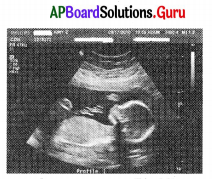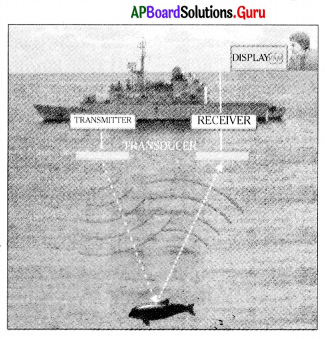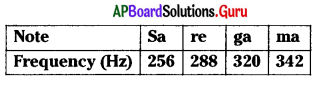Practice the AP 9th Class Physical Science Bits with Answers Chapter 11 Sound on a regular basis so that you can attempt exams with utmost confidence.

## AP State Syllabus 9th Class Physical Science Bits 11th Lesson Sound with Answers

Question 1.
Sound travels in the air in the form of
A) pulses
B) corpuscules
C) waves
D) particles
C) waves

Question 2.
Longitudinal waves involves change in …………………. of the medicine.
A) density
B) shape
C) velocity
D) temperature
A) densityQuestion 3.
Transverse waves involves change in ………………. of the medium.
A) density
B) shape
C) velocity
D) temperature
B) shape

Question 4.
Longitudinal wave of the following is
A) light wave
B) water wave
C) sound wave
D) none
C) sound wave

Question 5.
The maximum disturbance of particles in the medium on either side of mean position is called
A) wavelength
B) frequency
C) wave speed
D) amplitude
D) amplitude

Question 6.
The time taken for one oscillation is called
A) frequency
B) time period
C) wavelength
D) wave speed
B) time period

Question 7.
The matter or the substance through which sound is transmitted is called the …………………
A) object
B) medium
C) substance
D) quantity
B) medium

Question 8.
The distance between two consecutive compressions is ……………….
A) speed
B) wavelength
C) frequency
D) time period
B) wavelength

Question 9.
When a sound wave travels in air …………….. and ……………… of air at a place varies.
A) volume, density
B) pressure, volume
C) density, pressure
D) frequency, speed
C) density, pressureQuestion 10.
The regions where the density and pressure of particles is high are called as …………………
A) crusts
B) troughs
C) compressions
D) rarefactions
C) compressions

Question 11.
The regions where the density and pressure of particles is low are called as ………………….
A) crusts
B) troughs
C) compressions
D) rarefactions
D) rarefactions

Question 12.
Sound waves are ……………….. waves.
A) stationary
B) longitudinal
C) transverse
D) electromagnetic
B) longitudinal

Question 13.
If the particles of the medium vibrate along the direction of wave, then the wave is called a …………………..
A) longitudinal
B) transverse
C) stationary
D) electromagnetic
A) longitudinal

Question 14.
The unit of wavelength in S.l. system is …………………..
A) metre
B) hertz
C) decibel
D) m/s
A) metre

Question 15.
The unit of frequency of wave is ………………….
A) metre
B) hertz
C) decibel
D) second
B) hertz

Question 16.
Relation between time period (T) and frequency (υ) is …………………..
A) T = $$\frac{1}{υ}$$
B) υ = $$\frac{1}{\mathrm{~T}}$$
C) υ = T
D) Both A & B
D) Both A & B

Question 17.
Relation between speed of sound, frequency and wavelength is ………………
A) v = λυ
B) υ = vλ
C) λ = vυ
D) λvυ = 1
A) v = λυQuestion 18.
Speed of sound in water at 20°C = …………………
A) 330 m/s
B) 5500 m/s
C) 1484 m/s
D) 220 m/s
C) 1484 m/s

Question 19.
Speed of sound in air at 20°C is ……………….
A) 343.2 m/s
B) 1236 km/hr
C) 330 m/s
D) Both A & B
D) Both A & B

Question 20.
Pitch of sound depends on …………….
A) frequency
B) amplitude
C) wavelength
D) none of these
A) frequency

Question 21.
Loudness of sound depends on …………………..
A) frequency
B) amplitude
C) wavelength
D) none of these
B) amplitude

Question 22.
Reflection of sound will be better on ………………. surface than …………….. surface.
A) smooth, rough
B) rough, smooth
C) inclined, inverted
D) straight, inclined
B) rough, smooth

Question 23.
SONAR stands for …………………..
B) Sonographic Notation And Rating
C) Sound Notation And Rating

Question 24.
Region of low dense particles ………………….
A) compression
B) rarefaction
C) crust
D) trough
B) rarefactionQuestion 25.
Region of high dense particles is ………………
A) compression
B) rarefaction
C) crust
D) trough
A) compression

Question 26.
Number of oscillations in one second is called ……………………
A) wavelength
B) frequency
C) time period
D) amplitude
B) frequency

Question 27.
Maximum variation in the density is ………………
A) wavelength
B) frequency
C) time period
D) amplitude
D) amplitude

Question 28.
Distance between two consecutive compressions is …………………..
A) wavelength
B) frequency
C) time period
D) amplitude
A) wavelength

Question 29.
Sound is produced by
A) any body
B) vibrating body
C) a body in motion
D) bodies having different shapes
B) vibrating body

Question 30.
The characteristic of sound which distinguishes between a shrill sound and a growling sound is
A) pitch
B) loudness
C) quality
D) amplitude
A) pitch

Question 31.
Loudness of sound is measured in
A) Hertz
B) Meters
C) Decibels
D) Seconds
C) DecibelsQuestion 32.
The audible range of frequency for an average person is
A) 20 Hz – 200 Hz
B) 20 Hz – 20 kHz
C) 2 kHz – 20 kHz
D) 2000 Hz – 200 kHz
B) 20 Hz – 20 kHz

Question 33.
The loudness of sound is considered normal if it is between ………………
A) 50 dB to 60 dB
B) 80 dB
C) 20 dB
D) more than 120 dB
A) 50 dB to 60 dB

Question 34.
The decibel level of jet engine taking off is ……………..
A) 80 dB
B) 100 dB
C) 120 dB
D) 60 dB
C) 120 dB

Question 35.
Statement – I : If frequency of sound is less than 20 Hz, then they are called infrasonic sounds.
Statement – II : If frequency of sound is more than 20 Hz, then they are called infrasonic sounds.
A) Both the statements I & II are true.
B) Statement -1 is true and statement -II is false.
C) Statement -1 is false and statement – II is true.
D) Both the statements I & II are false.
A) Both the statements I & II are true.

Question 36.
Quality of a musical note depends on ……………….
A) instrument
B) wavelength
C) frequency
D) wave form
D) wave form

Question 37.
Sound of frequency less than 20 Hz is known as …………….. sound.
A) infrasonic
B) ultrasonic
C) echo
D) reverberation
A) infrasonicQuestion 38.
Sound of frequency greater than 20 kHz is known as ………………..
A) infrasonic
B) ultrasonic
C) echo
D) audible range
B) ultrasonic

Question 39.
The sounds heard by bat is upto …………….
A) 50 KHz
B) 25 KHz
C) 100 KHz
D) 5 KHz
C) 100 KHz

Question 40.
The maximum limit sounds heard by dog is ………………….
A) 50 KHz
B) 25 KHz
C) 100 KHz
D) 5 KHz
A) 50 KHz

Question 41.
The unit for intensity of sound is ……………….
A) hertz
B) metre
C) m/s
D) decibel
D) decibel

Question 42.
Adjacent picture is one of application ofA) Sound
B) Ultrasound
C) SONAR
D) All
B) Ultrasound

Question 43.
Adjacent picture represent the phenomenon ofA) Sound
B) Ultrasound
C) SONAR
D) All
C) SONAR

Question 44.
Echo is a reflected sound, reaches our ear, after direct sound in
A) lessthan 0.1s
B) more than 0.1s
C) exactly at 0.1s
D) any time
B) more than 0.1sQuestion 45.
Using ultrasounds in the imaging of organs like liver, uterus, etc. is called as
B) echography
C) ultrasonography
D) magnetic resonance imaging
C) ultrasonography

Question 46.
The location of under water objects can be determined by using
A) SONAR
B) Ultrasound
C) X-ray
D) Light waves
A) SONAR

Question 47.
A tabla and a guitar are played at a time. We can identify the sound from which instrument it is coming by the character of
A) pitch
B) loudness
C) quality
D) wave speed
C) quality

Question 48.
If the particles of the medium vibrate perpendicular to the direction of wave, then the wave is called a …………………
A) longitudinal
B) transverse
C) stationary
D) electromagnetic
B) transverse

Question 49.
As age increases, the audible range of frequency of sound …………………
A) increases
B) decreases
C) both A & B
D) none of these
B) decreases

Question 50.
While using SONAR, ……………….. can be utilised to determine the shape and size of the object.
A) refraction from various positions
B) reflection from various angles
C) both A & B
D) none of these
B) reflection from various angles

Question 51.
Which of the following is not a characteristic of musical sound ?
A) Wavelength
B) Pitch
C) Loudness
D) Quality
C) Loudness

Question 52.
Sound travels in a medium means
A) The medium travels
B) The particles of the medium travels
C) The sound travels
D) The disturbance travels
D) The disturbance travelsQuestion 53.Which Note has more pitch?
A) Sa
B) re
C) ga
D) ma
D) ma

Question 54
Identify the wrong pair among the following.
A) (Pitch, Frequency)
B) (Echo, Refraction)
C) (Quality, Waveform)
D) (Loudness, Amplitude)